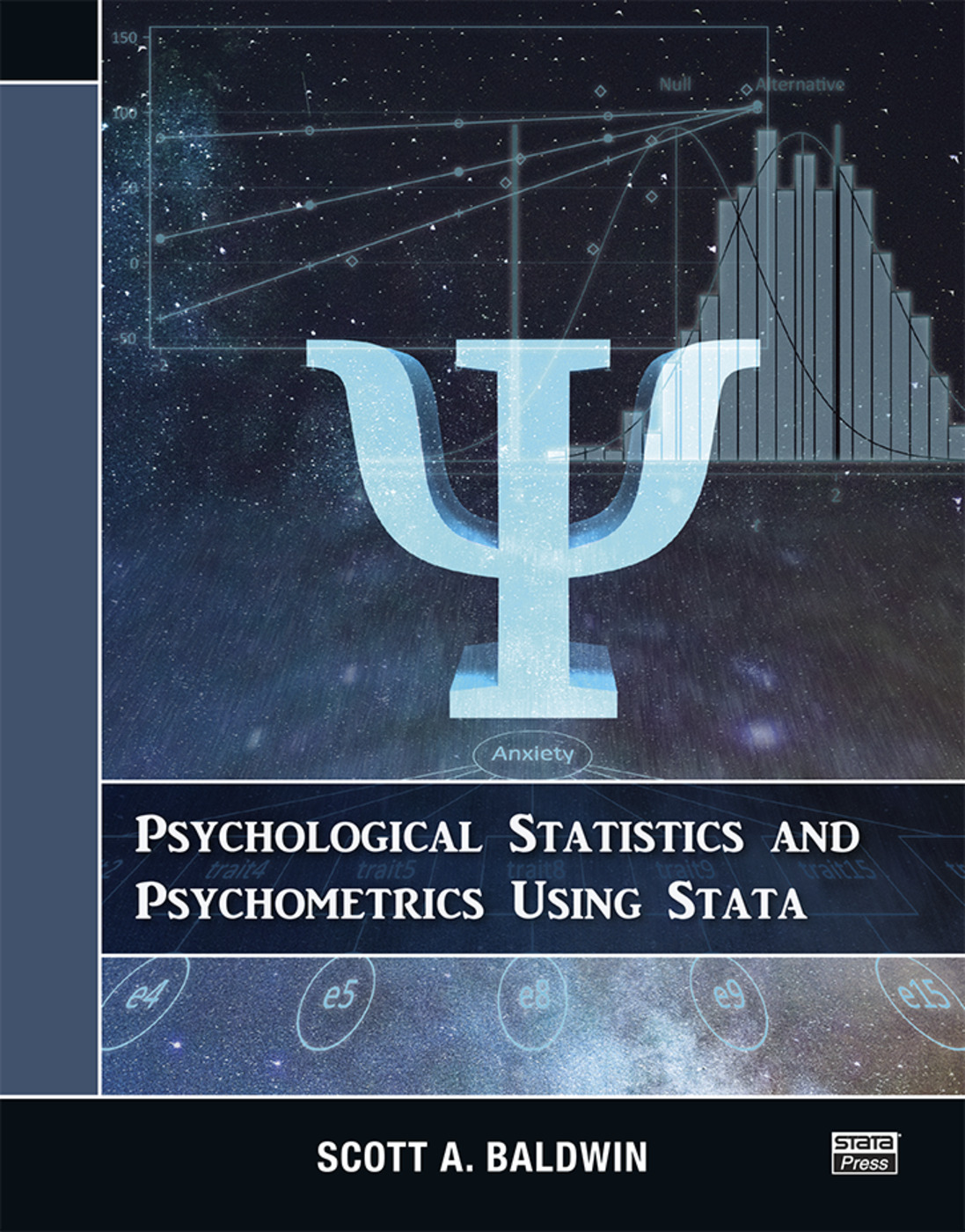Psychological Statistics and Psychometrics Using Stata

1st Edition

Stata Press

454 pages

Paperback: 9781597183031
pub: 2019-06-11
\$74.95
x

FREE Standard Shipping!

Description

Psychological Statistics and Psychometrics Using Stata is a complete and concise resource for students and researchers in the behavioral sciences. The author’s primary goal in this book is to help readers become competent users of statistics. To that end, he first introduces basic statistical methods such as regression, t tests, and ANOVA. He focuses on explaining the models, how they can be used with different types of variables, and how to interpret the results. After building this foundation, the author covers more advanced statistical techniques, including power-and-sample size calculations, multilevel modeling, and structural equation modeling. This book also discusses measurement concepts that are crucial in psychometrics. For instance, the author explores how reliability and validity can be understood and evaluated using exploratory and confirmatory factor analysis. The author includes dozens of worked examples using real data to illustrate the theory and concepts.

Notation and typography. Getting oriented to Stata. Introduction. Introduction to Stata. Understanding relationships between variables. Regression with continuous predictors. Regression with categorical and continuous predictors. t tests and one-way ANOVA. Factorial ANOVA. Repeated-measures models. Planning studies: Power and sample-size calculations. Multilevel models for cross-sectional data. Multilevel models for longitudinal data. Psychometrics through the lens of factor analysis. Factor analysis: Reliability. Factor analysis: Factorial validity. Measurement invariance. References.